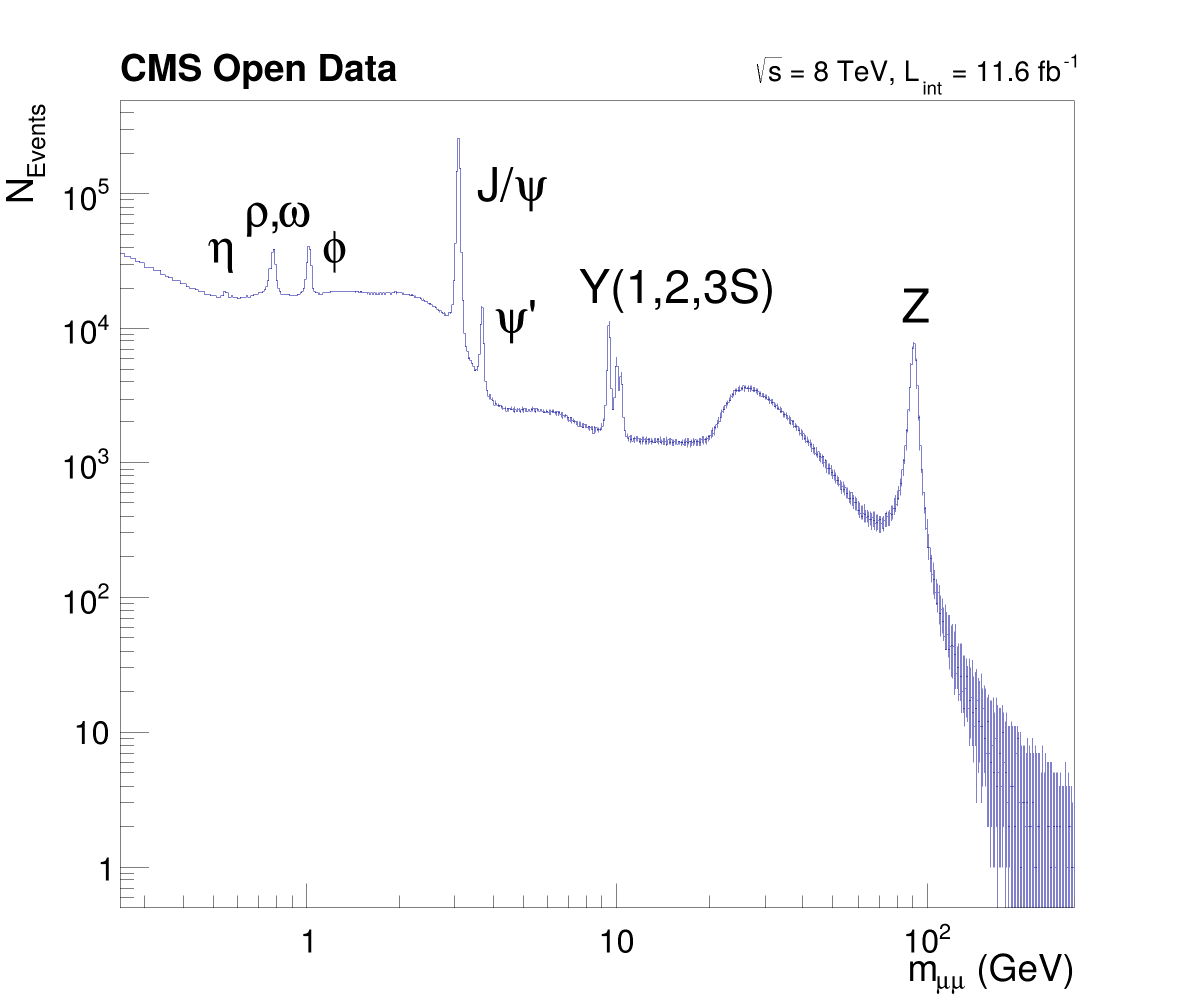ROOT   Reference Guidedf102_NanoAODDimuonAnalysis.py File Reference

## Namespaces

df102_NanoAODDimuonAnalysis

## Detailed DescriptionShow how NanoAOD files can be processed with RDataFrame.

This tutorial illustrates how NanoAOD files can be processed with ROOT dataframes. The NanoAOD-like input files are filled with 66 mio. events from CMS OpenData containing muon candidates part of 2012 dataset (DOI: 10.7483/OPENDATA.CMS.YLIC.86ZZ and DOI: 10.7483/OPENDATA.CMS.M5AD.Y3V3). The macro matches muon pairs and produces an histogram of the dimuon mass spectrum showing resonances up to the Z mass. Note that the bump at 30 GeV is not a resonance but a trigger effect.

More details about the dataset can be found on the CERN Open Data portal.import ROOT
# Enable multi-threading
ROOT.ROOT.EnableImplicitMT()
# Create dataframe from NanoAOD files
df = ROOT.RDataFrame("Events", "root://eospublic.cern.ch//eos/opendata/cms/derived-data/AOD2NanoAODOutreachTool/Run2012BC_DoubleMuParked_Muons.root")
# For simplicity, select only events with exactly two muons and require opposite charge
df_2mu = df.Filter("nMuon == 2", "Events with exactly two muons")
df_os = df_2mu.Filter("Muon_charge != Muon_charge", "Muons with opposite charge")
# Compute invariant mass of the dimuon system
df_mass = df_os.Define("Dimuon_mass", "InvariantMass(Muon_pt, Muon_eta, Muon_phi, Muon_mass)")
# Make histogram of dimuon mass spectrum. Note how we can set titles and axis labels in one go.
h = df_mass.Histo1D(("Dimuon_mass", "Dimuon mass;m_{#mu#mu} (GeV);N_{Events}", 30000, 0.25, 300), "Dimuon_mass")
# Request cut-flow report
report = df_mass.Report()
# Produce plot
ROOT.gStyle.SetOptStat(0); ROOT.gStyle.SetTextFont(42)
c = ROOT.TCanvas("c", "", 800, 700)
c.SetLogx(); c.SetLogy()
h.SetTitle("")
h.GetXaxis().SetTitleSize(0.04)
h.GetYaxis().SetTitleSize(0.04)
h.Draw()
label = ROOT.TLatex(); label.SetNDC(True)
label.DrawLatex(0.175, 0.740, "#eta")
label.DrawLatex(0.205, 0.775, "#rho,#omega")
label.DrawLatex(0.270, 0.740, "#phi")
label.DrawLatex(0.400, 0.800, "J/#psi")
label.DrawLatex(0.415, 0.670, "#psi'")
label.DrawLatex(0.485, 0.700, "Y(1,2,3S)")
label.DrawLatex(0.755, 0.680, "Z")
label.SetTextSize(0.040); label.DrawLatex(0.100, 0.920, "#bf{CMS Open Data}")
label.SetTextSize(0.030); label.DrawLatex(0.630, 0.920, "#sqrt{s} = 8 TeV, L_{int} = 11.6 fb^{-1}")
c.SaveAs("dimuon_spectrum.pdf")
# Print cut-flow report
report.Print()
Events with exactly two muons: pass=31104343 all=61540413 -- eff=50.54 % cumulative eff=50.54 %
Muons with opposite charge: pass=24067843 all=31104343 -- eff=77.38 % cumulative eff=39.11 %
Date
April 2019

Definition in file df102_NanoAODDimuonAnalysis.py.

ROOT::RDataFrame
ROOT's RDataFrame offers a high level interface for analyses of data stored in TTree,...
Definition: RDataFrame.hxx:42